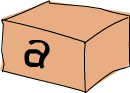# Types and Typeclasses

## Type``````ghci> :t 'a'
'a' :: Char
ghci> :t True
True :: Bool
ghci> :t "HELLO!"
"HELLO!" :: [Char]
ghci> :t (True, 'a')
(True, 'a') :: (Bool, Char)
ghci> :t 4 == 5
4 == 5 :: Bool
````````````removeNonUppercase :: [Char] -> [Char]
removeNonUppercase st = [ c | c <- st, c `elem` ['A'..'Z']]
``````

`removeNonUppercase` 的型别为 `[Char]->[Char]`，从它的参数和回传值的型别上可以看出，它将一个字串映射为另一个字串。`[Char]``String` 是等价的，但使用 `String` 会更清晰：`removeNonUppercase :: String -> String`。编译器会自动检测出它的型别，我们还是标明了它的型别声明。要是多个参数的函数该怎样？如下便是一个将三个整数相加的简单函数。

``````addThree :: Int -> Int -> Int -> Int
addThree x y z = x + y + z
``````

Int 表示整数。7 可以是 Int，但 7.2 不可以。Int 是有界的，也就是说它由上限和下限。对 32 位的机器而言，上限一般是 `2147483647`，下限是 `-2147483648`

Integer 表示...厄...也是整数，但它是无界的。这就意味着可以用它存放非常非常大的数，我是说非常大。它的效率不如 Int 高。

``````factorial :: Integer -> Integer
factorial n = product [1..n]
``````
``````ghci> factorial 50
30414093201713378043612608166064768844377641568960512000000000000
``````

Float 表示单精度的浮点数。

``````circumference :: Float -> Float
circumference r = 2 * pi * r
``````
``````ghci> circumference 4.0
25.132742
``````

Double 表示双精度的浮点数。

``````circumference' :: Double -> Double
circumference' r = 2 * pi * r
``````
``````ghci> circumference' 4.0
25.132741228718345
``````

Bool 表示布林值，它只有两种值：`True``False`

Char 表示一个字符。一个字符由单引号括起，一组字符的 List 即为字串。

Tuple 的型别取决于它的长度及其中项的型别。注意，空 Tuple 同样也是个型别，它只有一种值：`()`

## Type variables

``````ghci> :t head
````````````ghci> :t fst
fst :: (a, b) -> a
``````

## Typeclasses入门`==` 函数的型别声明是怎样的？

``````ghci> :t (==)
(==) :: (Eq a) => a -> a -> Bool
``````
``````*Note*: 判断相等的==运算子是函数，``+-*/``之类的运算子也是同样。在缺省条件下，它们多为中缀函数。若要检查它的型别，就必须得用括号括起使之作为另一个函数，或者说以首码函数的形式调用它。
``````

Eq 这一 Typeclass 提供了判断相等性的接口，凡是可比较相等性的型别必属于 `Eq` class。

``````ghci> 5 == 5
True
ghci> 5 /= 5
False
ghci> 'a' == 'a'
True
ghci> "Ho Ho" == "Ho Ho"
True
ghci> 3.432 == 3.432
True
``````

`elem` 函数的型别为: `(Eq a)=>a->[a]->Bool`。这是它在检测值是否存在于一个 List 时使用到了==的缘故。

Eq 包含可判断相等性的型别。提供实现的函数是 `==``/=`。所以，只要一个函数有Eq类的型别限制，那么它就必定在定义中用到了 `==``/=`。刚才说了，除函数以外的所有型别都属于 `Eq`，所以它们都可以判断相等性。

Ord 包含可比较大小的型别。除了函数以外，我们目前所谈到的所有型别都属于 `Ord` 类。`Ord` 包中包含了`<, >, <=, >=` 之类用于比较大小的函数。`compare` 函数取两个 `Ord` 类中的相同型别的值作参数，回传比较的结果。这个结果是如下三种型别之一：`GT, LT, EQ`

``````ghci> :t (>)
(>) :: (Ord a) => a -> a -> Bool
``````

``````ghci> "Abrakadabra" < "Zebra"
True
LT
ghci> 5 >= 2
True
ghci> 5 `compare` 3
GT
``````

Show 的成员为可用字串表示的型别。目前为止，除函数以外的所有型别都是 `Show` 的成员。操作 Show Typeclass，最常用的函数表示 `show`。它可以取任一Show的成员型别并将其转为字串。

``````ghci> show 3
"3"
ghci> show 5.334
"5.334"
ghci> show True
"True"
``````

Read 是与 `Show` 相反的 Typeclass。`read` 函数可以将一个字串转为 `Read` 的某成员型别。

``````ghci> read "True" || False
True
12.0
3
[1,2,3,4,3]
``````

``````ghci> read "4"
< interactive >:1:0:
Ambiguous type variable `a' in the constraint:
Probable fix: add a type signature that fixes these type variable(s)
``````

ghci 跟我们说它搞不清楚我们想要的是什么样的回传值。注意调用 `read` 后跟的那部分，ghci 通过它来辨认其型别。若要一个 `boolean` 值，他就知道必须得回传一个 `Bool` 型别的值。但在这里它只知道我们要的型别属于 Read Typeclass，而不能明确到底是哪个。看一下 `read` 函数的型别声明吧：

``````ghci> :t read

5
5.0
ghci> (read "5" :: Float) * 4
20.0
[1,2,3,4]
ghci> read "(3, 'a')" :: (Int, Char)
(3, 'a')
``````

Enum 的成员都是连续的型别 -- 也就是可枚举。`Enum` 类存在的主要好处就在于我们可以在 `Range` 中用到它的成员型别：每个值都有后继子 (successer) 和前置子 (predecesor)，分别可以通过 `succ` 函数和 `pred` 函数得到。该 Typeclass 包含的型别有：`()`, `Bool`, `Char`, `Ordering`, `Int`, `Integer`, `Float``Double`

``````ghci> ['a'..'e']
"abcde"
ghci> [LT .. GT]
[LT,EQ,GT]
ghci> [3 .. 5]
[3,4,5]
ghci> succ 'B'
'C'
``````

Bounded 的成员都有一个上限和下限。

``````ghci> minBound :: Int
-2147483648
ghci> maxBound :: Char
'\1114111'
ghci> maxBound :: Bool
True
ghci> minBound :: Bool
False
``````

`minBound``maxBound` 函数很有趣，它们的型别都是 `(Bounded a) => a`。可以说，它们都是多态常量。

``````ghci> maxBound :: (Bool, Int, Char)
(True,2147483647,'\1114111')
``````

Num 是表示数字的 Typeclass，它的成员型别都具有数字的特征。检查一个数字的型别：

``````ghci> :t 20
20 :: (Num t) => t
``````

``````ghci> :t (*)
(*) :: (Num a) => a -> a -> a
``````

Integral 同样是表示数字的 Typeclass。`Num` 包含所有的数字：实数和整数。而 Integral 仅包含整数，其中的成员型别有 `Int``Integer`

Floating 仅包含浮点型别：`Float``Double`